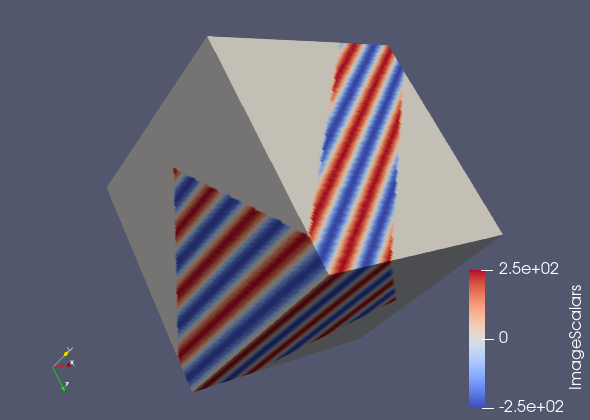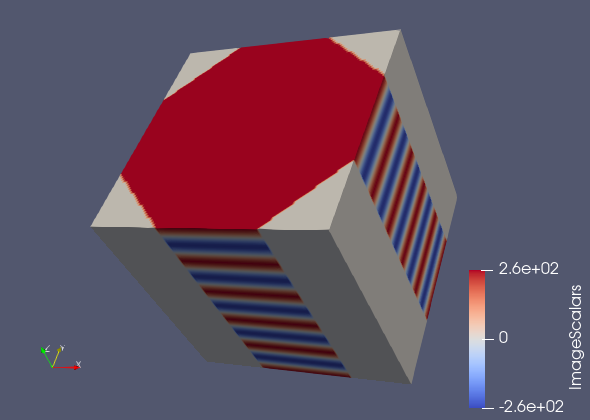# Help with vtkImageReslice

I am trying to generate oblique slices through image data - and I think that I’m starting out with a simple example. I generated a cube with a sinusoidal pattern in it, and I wanted to slice through the center of the cube.

I tried to set the sinusoid source up so that it generates waves travelling in the (1, 1, 1)/sqrt(3) direction. I then tried to get a slice through the center of the cube perpendicular to that direction - so that I would get a plane that has all one color. The output was a bit surprising to me - it wasn’t a plane, it was thick, but it seemed jagged somehow as well.

``````import vtk

s = vtk.vtkImageSinusoidSource()
s.SetDirection(1, 1, 1)
s.SetWholeExtent(0, 100, 0, 100, 0, 100)
s.Update()

r = vtk.vtkImageReslice()

a = 1/np.sqrt(2)
b = 1/np.sqrt(3)

r.SetResliceAxesDirectionCosines(
a, a, 0,
-a, a, 0,
b, b, b)
r.SetResliceAxesOrigin(50, 50, 50)
r.SetInputConnection(s.GetOutputPort())

w = vtk.vtkXMLImageDataWriter()
w.SetInputConnection(r.GetOutputPort())
w.SetFileName('hi.vti')
w.Write()
``````Ok - I think I am beginning to understand how this works. I edited the script to have an axis-aligned wavefront and a simpler rotation. The first image was a projection of sorts through the original image.

Something important I missed while reading the documentation: “The method SetOutputDimensionality(2) is used to specify that want to output a slice rather than a volume.” But I still am not sure how to set the thickness of that slice - if that is a possibility.

``````s = vtk.vtkImageSinusoidSource()
s.SetDirection(0, 0, 1)
s.SetWholeExtent(0, 100, 0, 100, 0, 100)
s.Update()

r = vtk.vtkImageReslice()

a = 1/np.sqrt(2)
b = 1/np.sqrt(3)

r.SetResliceAxesDirectionCosines(
np.cos(a), np.sin(a), 0,
-np.sin(a), np.cos(a), 0,
0, 0, 1)

r.SetResliceAxesOrigin(50, 50, 50)
r.SetInputConnection(s.GetOutputPort())

w = vtk.vtkXMLImageDataWriter()
w.SetInputConnection(r.GetOutputPort())
w.SetFileName('hi.vti')
w.Write()
``````To get you to the next step, you have to set the OutputExtent for vtkImageReslice:

``````r.SetOutputExtent(0, 100, 0, 100, 0, 0)
``````

If you do this, you should get a single slice as output. Note that, in VTK and ParaView, a single image slice has zero thickness. The thickness of an image slice is the same as the thickness of a polygon, which is also zero.

If you want to impart some thickness for the sake of visualization, then 2 slices can be used:

``````r.SetOutputExtent(0, 100, 0, 100, 0, 1)
``````

I know this isn’t the same as one thick slice, but VTK doesn’t have any way of showing a single slice as anything other than two-dimensional.

Also note: the default behavior of vtkImageReslice is to place the center of the output volume (or slice) at the center of the input volume. This makes it easy to pick out the middle slice of a volume, or to simply reslice the whole volume from a different angle.

Selecting slices other than the middle slice requires calling `reslice.SetOutputOrigin()`. And that’s where things start getting complicated, because this origin is the corner of the output volume (or slice), and when the coordinate system is rotated, it’s not immediately obvious where the corner of the output volume (or slice) should be in order to get a specific slice of the input volume.

Thanks, that was the help I needed!